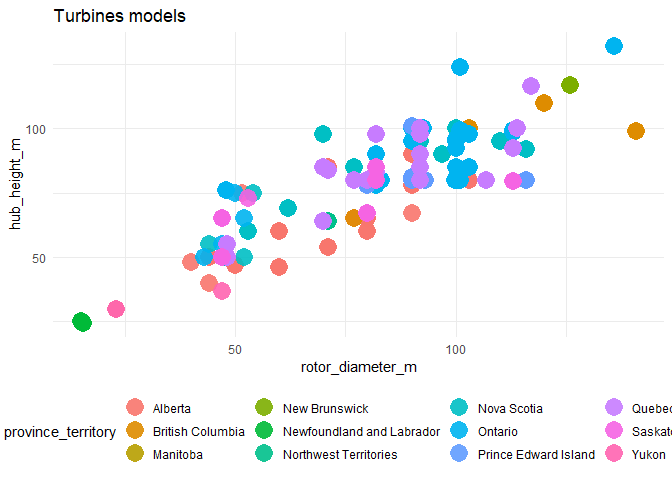# TidyTuesday 2020-10-27

If you want to join the next CorrelAid TidyTuesday Meetup, make sure to sign up to our Newsletter or reach out to us on Twitter!

## Some pre-meetup inspiration and tips & tricks

By Cédric Scherer

``````library(tidyverse)
library(tidytuesdayR)
library(ragg)

theme_set(theme_minimal(base_family = "Roboto Condensed"))

``````
``````##
``````
``````df_wind <- tt\$`wind-turbine`
``````

You can quickly build a bar plot with `geom_bar(aes(x))`:

``````ggplot(df_wind, aes(x = province_territory)) +
geom_bar() +
coord_flip()
``````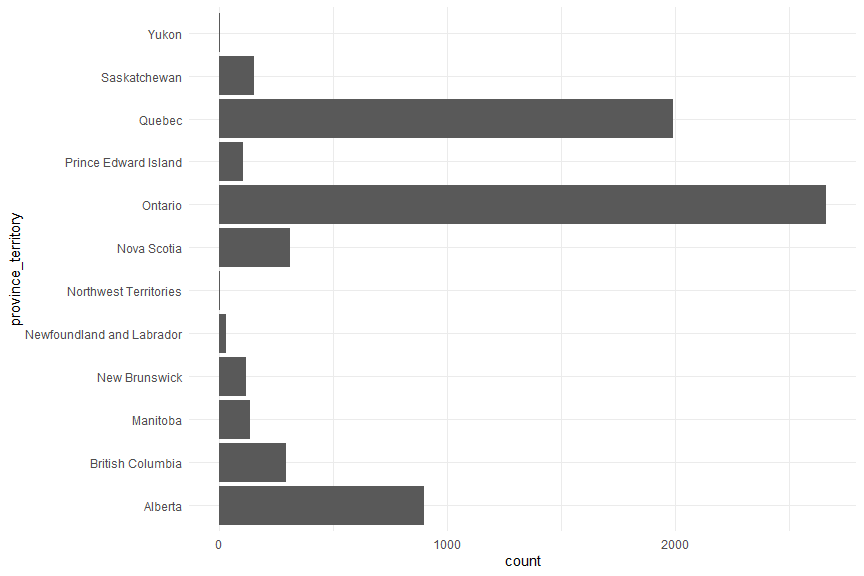Actually I prefer to caclulate the summaries first, then one uses `geom_col(aes(x, y))`. One good thing about it: You can easily sort the bars in an increasing order for better readability:

``````df_wind %>%
count(province_territory) %>%
ggplot(aes(forcats::fct_reorder(province_territory, n), n)) +
geom_col() +
coord_flip()
``````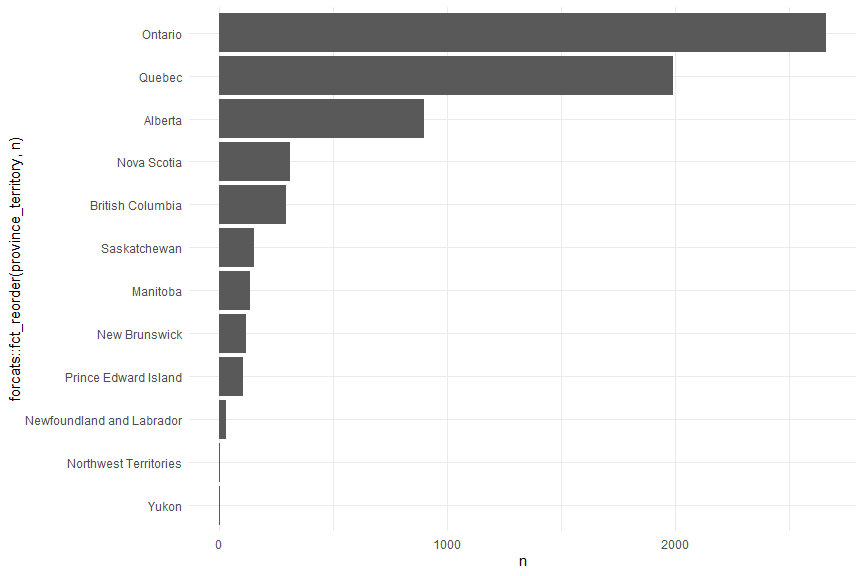This way, one can also quickly filter by the number of wind turbines to focus on the most comon categories:

``````df_wind %>%
count(project_name) %>%
filter(n > 50) %>%
ggplot(aes(forcats::fct_reorder(project_name, n), n)) +
geom_col() +
coord_flip()
``````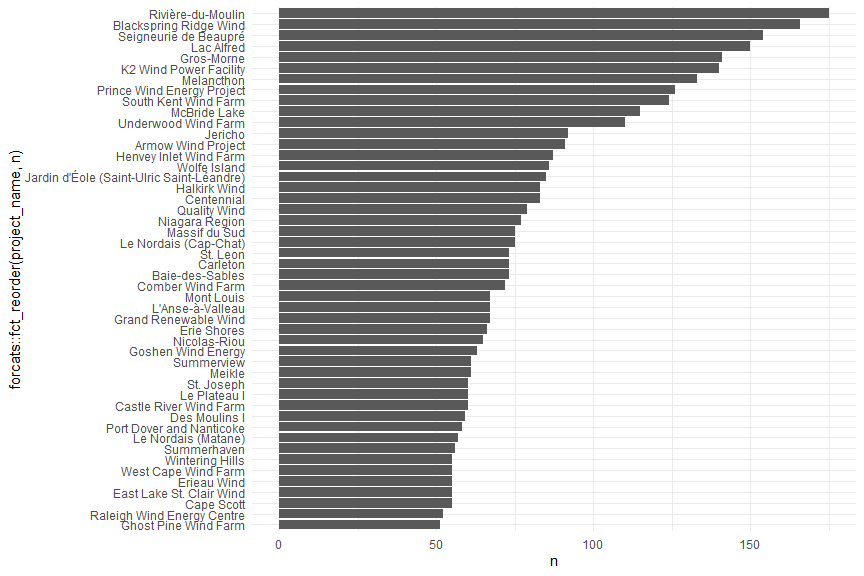If you want to produce a map, turn the dataframe into an spatial object (preferably an `sf` object) and plot it afterwards with `geom_sf()`. Note that you don’t need to specify `x` and `y`—ggplot’s `geom_sf()` wnows that the coords are what it needs to plot.

Why not simply plot longitude versus latitude? Yes, you can do that but if you want to add a map underneath it needs to hvae the same projection, otherwise your points will not match with the map. Turning the data into a spatial object allows to reproject (change the projection of/transform) the data, e.g. into the Lambert Conformal Conic projection that is often used for Canada and the US:

``````library(sf)

sf_wind <-
df_wind %>%
st_as_sf(coords = c("longitude", "latitude"),
crs = "+proj=longlat +datum=WGS84 +no_defs") %>%
st_transform(crs = "+proj=lcc +lon_0=-90 +lat_1=33 +lat_2=45")

rnaturalearth::ne_countries(scale = 110, country = "Canada",
returnclass = "sf") %>%
st_transform(crs = st_crs(sf_wind))

geom_sf(color = NA, fill = "grey92") +
geom_sf(data = sf_wind, color = "#1D994E", alpha = .1,
size = 2, shape = 21, fill = NA)
``````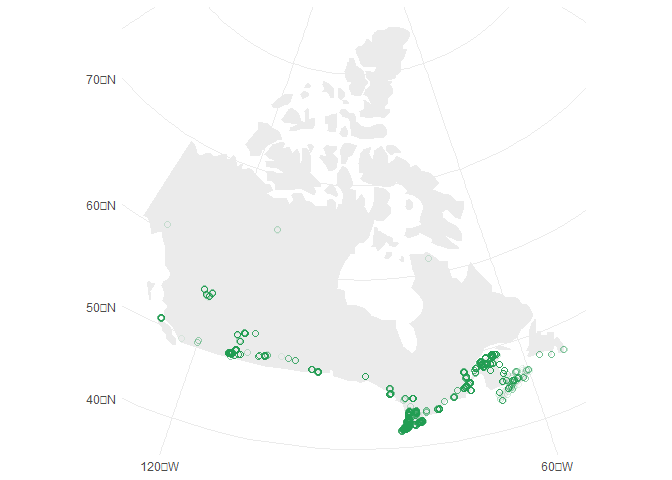Density maps are a quick solution to deal with oveprlotting (as it’s the case here). It is very simple to make a hex-bin density map in one line with `geom_hex()`:

``````ggplot(df_wind, aes(longitude, latitude)) +
geom_hex()
``````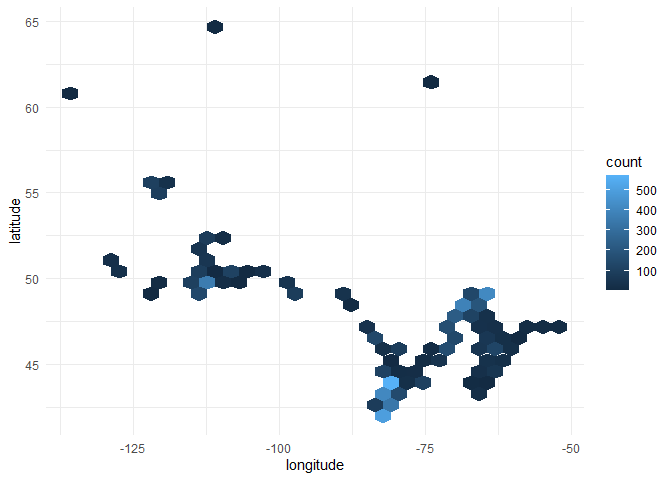### Tips’n’Tricks:

• `GGally::ggpairs()` for a quick EDA
• `rnaturalearth::ne_countries()` and `rnaturalearth::ne_states()` for shape files of countries
• `{ggforce}` package for many many cool things in ggplot2
• `forcats::fct_reorder()` and `forcats::fct_lump()` to reorder factors based on variables or merge those of low interest into a “other” class
• `theme(plot.title.position = "plot", plot.caption.position = "plot")` since `{ggplot2}` v3.0.0 to justify the title, subtitle and caption with the plot area not the panel border
• Type sorted list of color palettes in R

## A beautiful viz integrating data and the canadian flag

By Cédric Scherer

You can find the code on Cédrics GitHub.

#TidyTuesday Week 2020/44 🇨🇦 Wind Turbines in @Canada I like #maps.I like #flags.#r4ds #rstats #tidyverse #rspatial #mapping #dataviz #datavis #DataVisualization pic.twitter.com/M7zS2DqeHj

— Cédric Scherer (@CedScherer) October 28, 2020

## An animation showing the turbines being built over the years

By Long Nguyen

``````library(tidyverse)
library(sf)
library(rnaturalearth)
library(gganimate)
library(rgeos)
library(rnaturalearthhires) # install with devtools::install_github("ropensci/rnaturalearthhires")
``````
``````wind_turbine_welp <- wind_turbine %>%
mutate(commissioning_date_welp = str_sub(commissioning_date, end = 4) %>%
as.integer())
anim_turbines <- ne_states("Canada", returnclass = "sf") %>%
ggplot() +
geom_sf(colour = "#f8f8f8") +
geom_jitter(data = wind_turbine_welp,
aes(longitude, latitude, colour = turbine_rated_capacity_k_w,
group = seq_along(objectid)),
alpha = .4, size = 2.5) +
scale_colour_viridis_c(
begin = .3, end = .95, direction = -1,
labels = function(x) paste(x, "kW"),
guide = guide_legend(override.aes = list(alpha = 1, size = 2.5))
) +
labs(title = "Wind turbines in Canada",
subtitle = "Year: {frame_along}") +
theme_void(base_family = "Asap Condensed", base_size = 16) +
theme(legend.position = "bottom", legend.title = element_blank()) +
transition_reveal(commissioning_date_welp)
# animate(anim_turbines, end_pause = 6, width = 600, height = 600, units = "px") # commented out for knitting
# gganimate::anim_save(here::here("2020-10-27/wind_turbines_over_years.gif")) # commented out for knitting
``````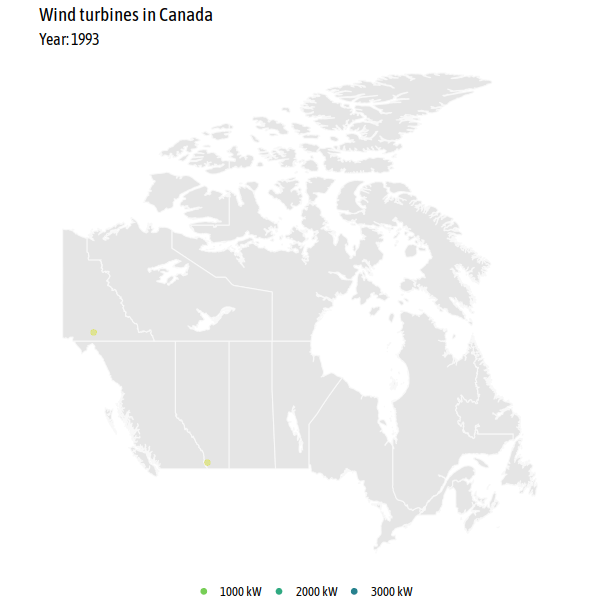## Number of wind turbines in the three most-wind-focused provinces

By Lisa Reiber

``````library(tidyverse)
library(ggplot2)
library(emoGG)
``````
``````witu %>%
drop_na(project_name) %>%
drop_na(province_territory) %>%
count(project_name, province_territory) %>%
mutate(project_name_f = fct_reorder(project_name, n),
project_name_trunc = fct_lump_min(project_name_f, min = 50, w = n),
province_trunc = fct_collapse(province_territory,
Ontario = "Ontario",
Quebec = "Quebec",
Alberta = "Alberta",
other_level = "All Other Provinces")
) %>%
filter(n > 15) %>%
ggplot(aes(project_name_f, n, group = province_trunc, label = project_name)) +
geom_col(width = 0.3) +
emoGG::geom_emoji(emoji = "1f341") + #canada leaf
facet_grid(province_trunc ~.,
scales = "free_x",
space = "free",
switch = "y"
) +
theme_light() +
theme(plot.title.position = "plot", #so cool <3
axis.text.x = element_blank(),
axis.ticks.x = element_blank(),
panel.grid.major = element_blank()
) +
labs(y = "",
x = "",
title = "Comparing Wind-Turbine Projects in Alberta, Ontario and Quebec to the rest of Canada",
subtitle = "for projects with more than 15 turbines"
) +
scale_fill_grey() +
scale_y_continuous(expand = c(0, 0))
``````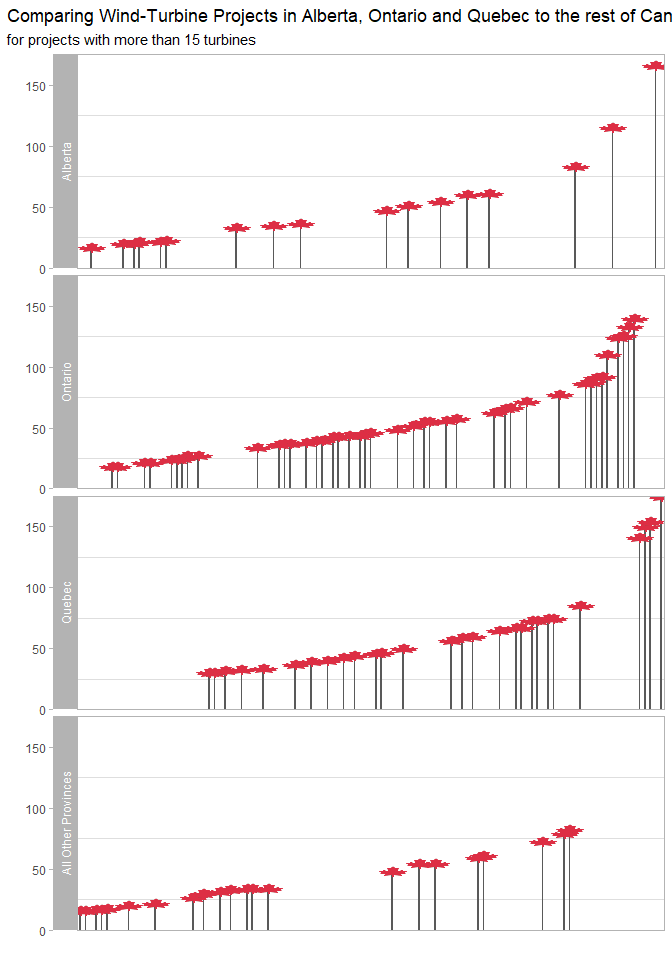``````      NULL
``````
``````## NULL
``````

## An interactive map with 3D wind turbines

By Frie Preu

``````library(tidytuesdayR)
library(sf)
library(tidyverse)
library(mapdeck)

key <- Sys.getenv("MAPBOX_TOKEN") # you can create a mapbox token for free after registering at https://www.mapbox.com/

``````
``````##
``````
``````turbines <- tt\$`wind-turbine`
``````

#### Create tooltip to use later

``````# tooltip for turbines
turbines <- turbines %>%
mutate(tooltip = glue::glue("{turbine_identifier}: commissioned {commissioning_date} as part of project {project_name} \n Hub height (m): {hub_height_m} \n Rotor diameter (m): {rotor_diameter_m} \n capacity: {turbine_rated_capacity_k_w} kw"))
``````

``````# uncomment the following lines to download and unzip
# url <- "http://www12.statcan.gc.ca/census-recensement/2011/geo/bound-limit/files-fichiers/gpr_000b11a_e.zip"

turbines_sf <- sf::st_as_sf(turbines, coords = c("longitude", "latitude"), crs = 4269)
``````

#### Calculate province level stats

``````# this could probably be one pipe but for easier understandability they are two :)
# total capacity (project) by province
province_capacities <- turbines %>%
group_by(project_name) %>%
mutate(capacity = mean(total_project_capacity_mw)) %>%
distinct(province_territory, project_name, capacity) %>% # dirty
group_by(province_territory) %>%
summarize(sum_capacity = round(sum(capacity), 2))

# number of turbines and number of projects
province_ns <- turbines %>%
group_by(province_territory) %>%
summarize(n_projects = n_distinct(project_name),
n_turbines = n())

# join the two datasets
province_data <- left_join(province_ns, province_capacities, by = "province_territory")

# create tooltip that is later used in mapdeck
province_data <- province_data %>%
mutate(tooltip = glue::glue("{province_territory}: &#013;&#010; #turbines: {n_turbines} &#013;&#010; #projects: {n_projects} &#013;&#010; total project capacity (MW): {sum_capacity}"))
``````

#### Join spatial datasets with calculated data

``````# this could probably be done way more elegantly with better knowledge of sf!
# join the data to the spatial file, and then join the polygons with the points
turbines_sf <- left_join(turbines_sf, province_data, by = "province_territory")

# "aggregate" turbine spatial data to get the PRUID and province_territory variables in a province level dataset
sum(is.na(canada_turbines\$PRUID)) # 4 turbines are not associated to a polygon
``````
``````##  4
``````
``````province_data_with_uid <- canada_turbines %>%
filter(!is.na(PRUID)) %>%
st_drop_geometry() %>%
distinct(PRUID, province_territory, n_projects, n_turbines, sum_capacity)

# join the province data with the province_territory and the data to the spatial canada data

# create tooltip for provinces
mutate(tooltip = glue::glue("{province_territory}: &#013;&#010; #turbines: {n_turbines} &#013;&#010; #projects: {n_projects} &#013;&#010; total project capacity (MW): {sum_capacity}"))
``````

#### Mapdeck!

``````m <- mapdeck(token = key, style = mapdeck_style("dark"), pitch = 45 ) %>%
fill_colour = "sum_capacity",
fill_opacity = 0.4,
tooltip = "tooltip",
palette = "ylgn",
layer_id = "provinces",
legend = TRUE,
legend_options = list(
fill_colour = list( title = "Total capacity (MW)"))
) %>%
lat = "latitude",
lon = "longitude",
fill_colour = "#C0392BFF",
elevation = "hub_height_m",
elevation_scale = 1000,
layer_id = "turbines",
tooltip = "tooltip"
)
# m # commented out for knitting
``````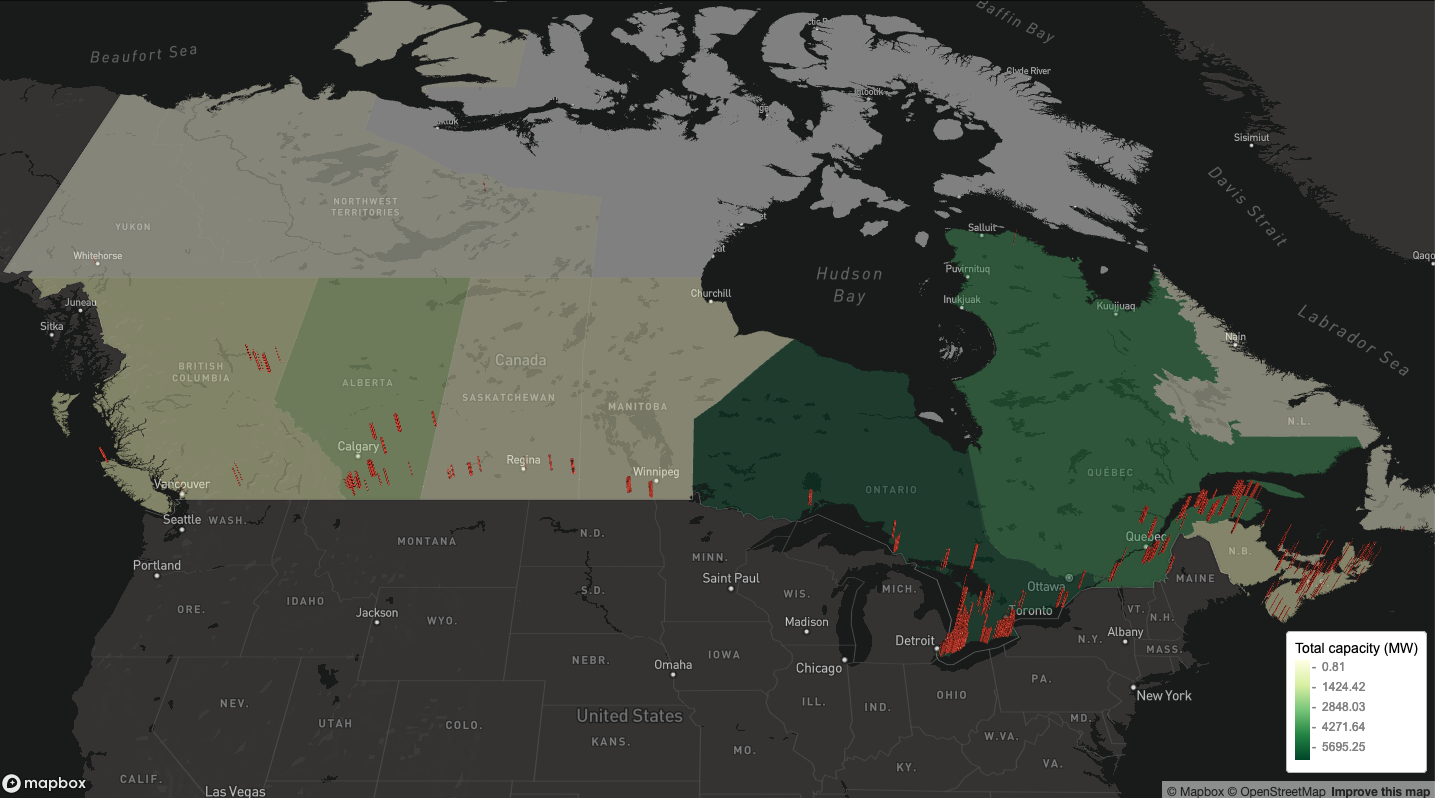The interactive version of the map can be found here

## A (WIP) animation of newly added wind turbines over the years

By Andreas Neumann

``````library(downloader)
library(tmap)
library(sf)
###data wind turbines###

names(Wind_Turbine_Database_FGP) <- "territory"
names(Wind_Turbine_Database_FGP) <- "year"

Wind_Turbine_Database_FGP\$year <- as.numeric(Wind_Turbine_Database_FGP\$year)
df <- Wind_Turbine_Database_FGP %>%
dplyr::group_by(territory, year) %>%
dplyr::summarise(Freq = n())

``````
``````## Reading layer `canada_provinces' from data source `C:\Users\DataVizard\Google Drive\Work\Programing\R\correlaid-tidytuesday\2020-10-27\canada_provinces\canada_provinces\canada_provinces.geojson' using driver `GeoJSON'
## Simple feature collection with 13 features and 7 fields
## geometry type:  MULTIPOLYGON
## dimension:      XY
## bbox:           xmin: -141.0181 ymin: 41.7297 xmax: -52.6194 ymax: 74
``````
``````names(canada) <- "territory"
###Merge the data and create maps###
crs = "+datum=WGS84")
carto_norm = carto %>%
split(.\$year) %>%
do.call(rbind, .)
``````
``````anim_can = tm_shape(canada) + tm_polygons(col = "lightblue") + tm_shape(carto_norm) +
tm_polygons("Freq", title = "windmillcount: ") +
tm_facets(along = "year",
free.coords = FALSE,
drop.units = TRUE) +
tm_layout(legend.outside.position = "right",
legend.outside = TRUE) + tm_text("Freq")
# tmap_animation(
#   anim_can,
#   delay = 150,
#   width = 1326,
#   height = 942
# ) # commented out for knitting
``````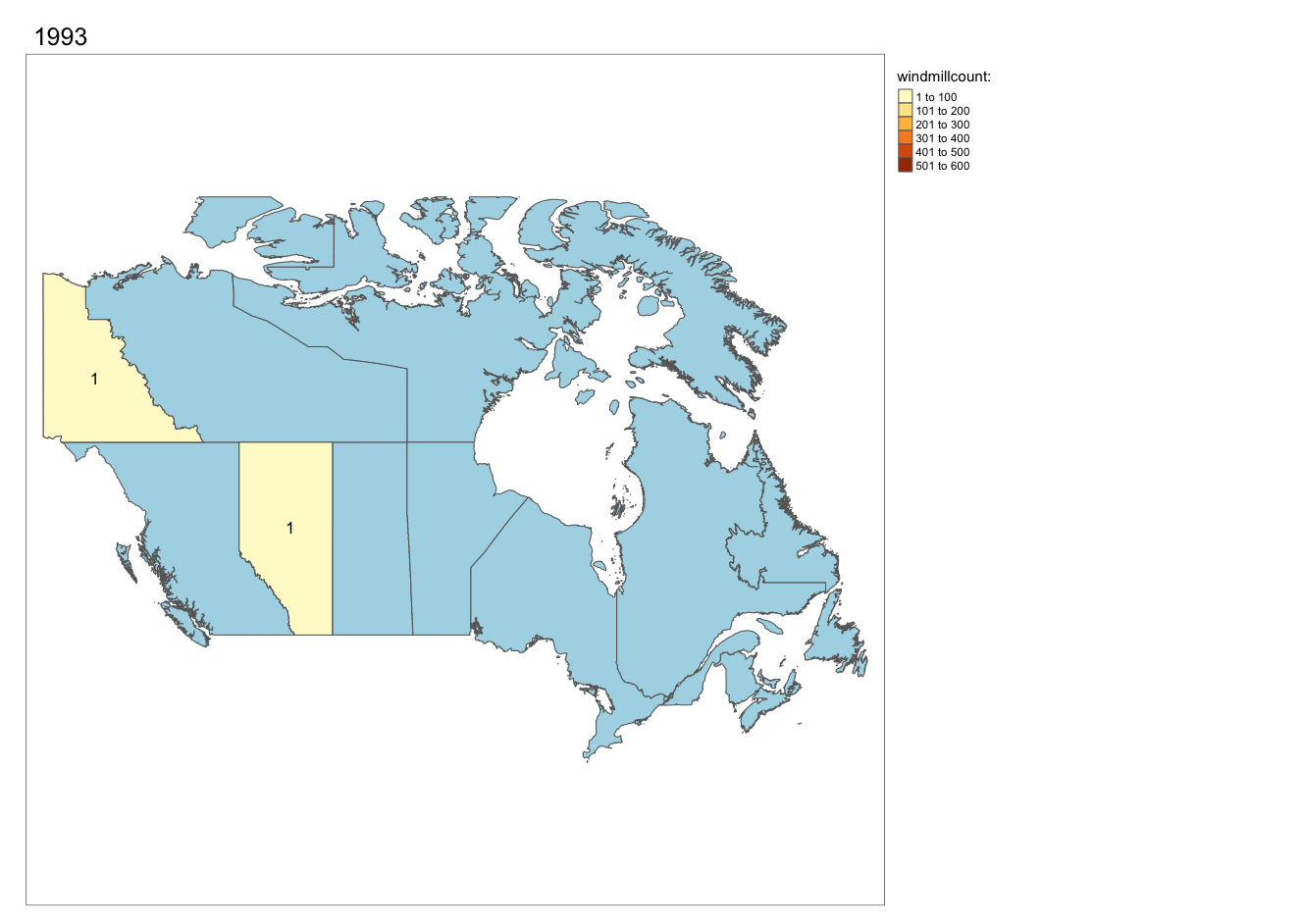## Do manufactureres have a province “preference”?

By Sylvi Rzepka

``````library(ggplot2)
library(dplyr)
library(viridis)
wind2 <- wind_turbine %>%
filter(
province_territory == "Alberta" |
province_territory == "Ontario" |
province_territory == "Quebec"
) %>%
mutate(manufacturer_fct = as.factor(manufacturer)) %>%
mutate(manufacturer_fct_o = fct_lump_min(manufacturer_fct, 19)) %>%
count(province_territory, manufacturer_fct_o)

ggplot(wind2,
aes(fill = manufacturer_fct_o, x = n, y = province_territory)) +
geom_bar(stat = "identity") +
labs(
x = NULL,
y = "Province",
color = NULL,
title = "Manufacturers by Province"
) +
scale_fill_viridis(discrete = T, direction = -1) +
theme_minimal() +
theme(legend.title = element_blank(),
legend.position = "bottom")
``````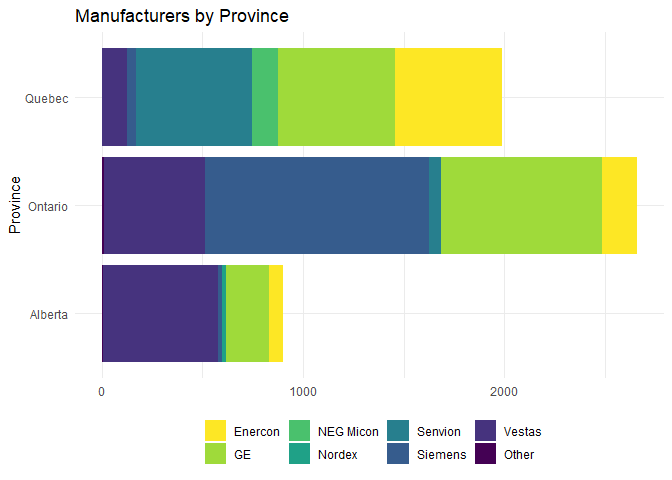## A classic bar plot

By Daniela Vogler

``````library(dplyr)
library(ggplot2)
library(stringr)
``````
``````wt_height <- wind_turbine  %>%
mutate(commissioning_date = as.numeric(str_sub(commissioning_date, start=1, end=4))) %>%
group_by(commissioning_date) %>%
summarize(avg_hub_height = mean(hub_height_m))
ggplot(wt_height) +
geom_col(aes(x = commissioning_date, y = avg_hub_height)) +
labs(title = "Height of Wind Turbines", x = "Year", y = "Average Height (m)") +
theme_classic()
``````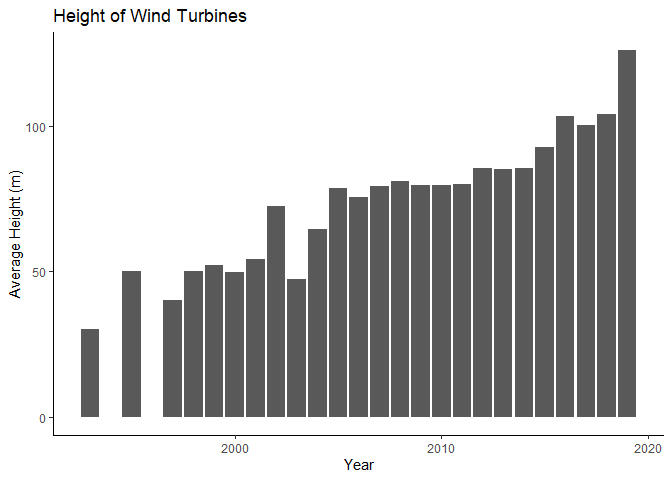## A colorful scatterplot

By Heba Azzam

``````library(ggplot2)
``````wind_turbine %>% ggplot(aes(x= rotor_diameter_m, y= hub_height_m, color= province_territory)) +